Next: Optical Theorem Up: Scattering Theory Previous: Born Approximation

# Partial Waves

We can assume, without loss of generality, that the incident wavefunction is characterized by a wavevector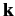that is aligned parallel to the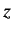-axis. The scattered wavefunction is characterized by a wavevector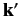that has the same magnitude as, but, in general, points in a different direction. The direction ofis specified by the polar angle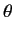(i.e., the angle subtended between the two wavevectors), and an azimuthal angleabout the-axis. Equation (943) strongly suggests that for a spherically symmetric scattering potential [i.e.,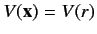] the scattering amplitude is a function ofonly: i.e.,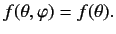(955)

It follows that neither the incident wavefunction,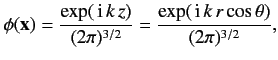(956)

nor the total wavefunction,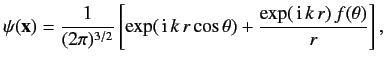(957)

depend on the azimuthal angle.

Outside the range of the scattering potential, both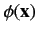and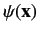satisfy the free space Schrödinger equation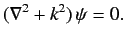(958)

Consider the most general solution to this equation in spherical polar coordinates that does not depend on the azimuthal angle. Separation of variables yields(959)

since the Legendre polynomials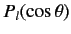form a complete set in-space. The Legendre polynomials are related to the spherical harmonics introduced in Chapter 4 via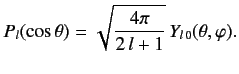(960)

Equations (958) and (959) can be combined to give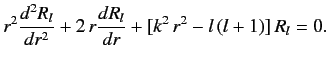(961)

The two independent solutions to this equation are the spherical Bessel function,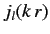, and the Neumann function,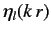, where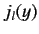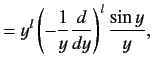(962)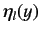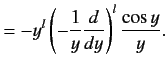(963)

Note that spherical Bessel functions are well-behaved in the limit, whereas Neumann functions become singular. The asymptotic behaviour of these functions in the limit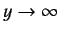is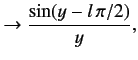(964)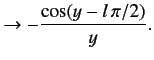(965)

We can write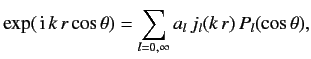(966)

where the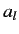are constants. Note there are no Neumann functions in this expansion, because they are not well-behaved as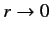. The Legendre polynomials are orthogonal,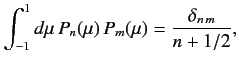(967)

so we can invert the above expansion to give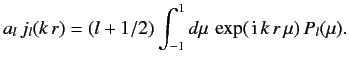(968)

It is well-known that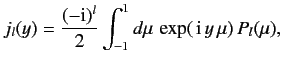(969)

where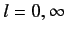. Thus,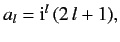(970)

giving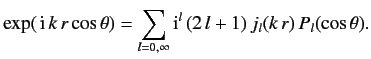(971)

The above expression tells us how to decompose a plane wave into a series of spherical waves (or partial waves'').

The most general solution for the total wavefunction outside the scattering region is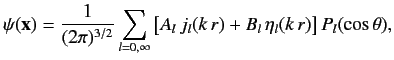(972)

where the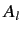and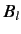are constants. Note that the Neumann functions are allowed to appear in this expansion, because its region of validity does not include the origin. In the large-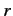limit, the total wavefunction reduces to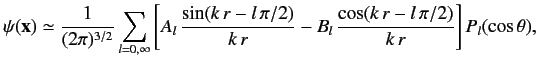(973)

where use has been made of Equations (964)-(965). The above expression can also be written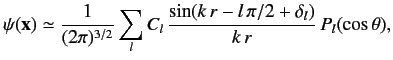(974)

where the sine and cosine functions have been combined to give a sine function that is phase-shifted by.

Equation (974) yields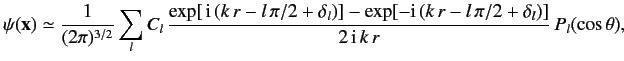(975)

which contains both incoming and outgoing spherical waves. What is the source of the incoming waves? Obviously, they must be part of the large-asymptotic expansion of the incident wavefunction. In fact, it is easily seen that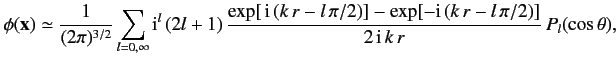(976)

in the large-limit. Now, Equations (956) and (957) give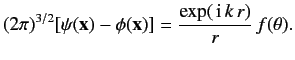(977)

Note that the right-hand side consists only of an outgoing spherical wave. This implies that the coefficients of the incoming spherical waves in the large-expansions ofandmust be equal. It follows from Equations (975) and (976) that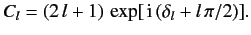(978)

Thus, Equations (975)-(977) yield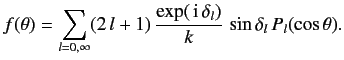(979)

Clearly, determining the scattering amplitude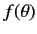via a decomposition into partial waves (i.e., spherical waves) is equivalent to determining the phase-shifts.Next: Optical Theorem Up: Scattering Theory Previous: Born Approximation
Richard Fitzpatrick 2013-04-08